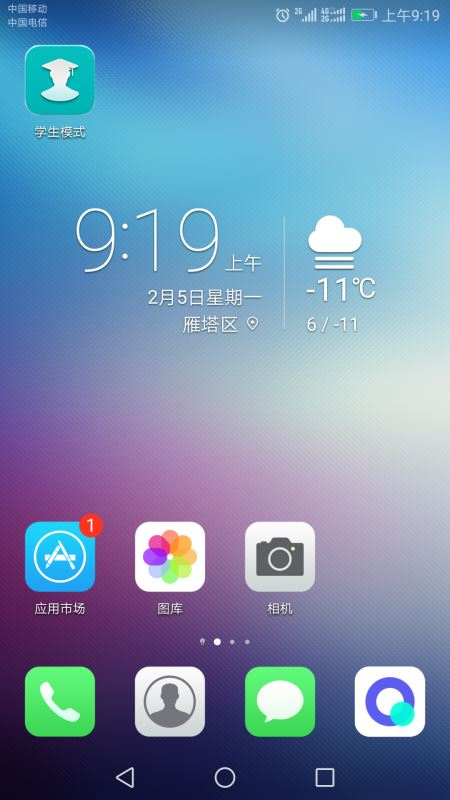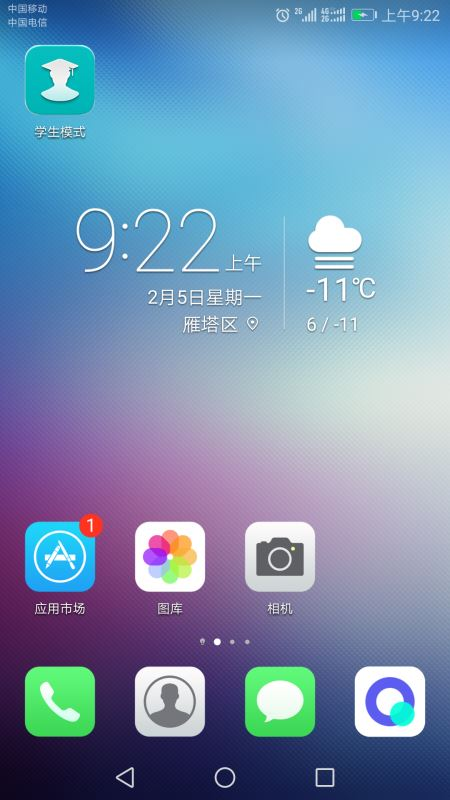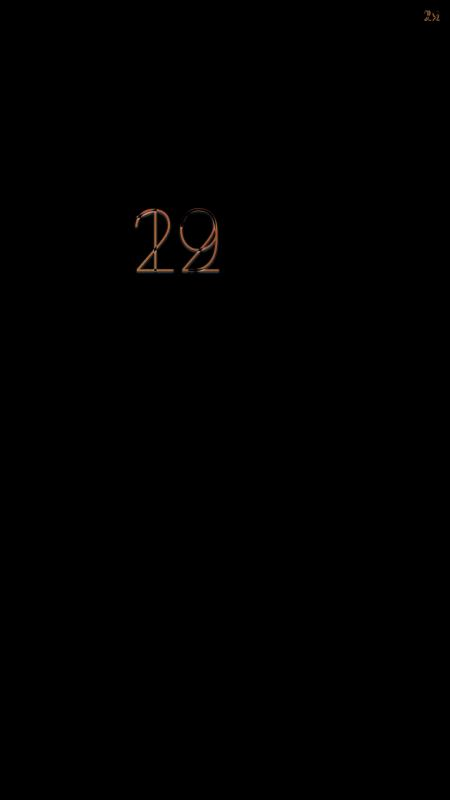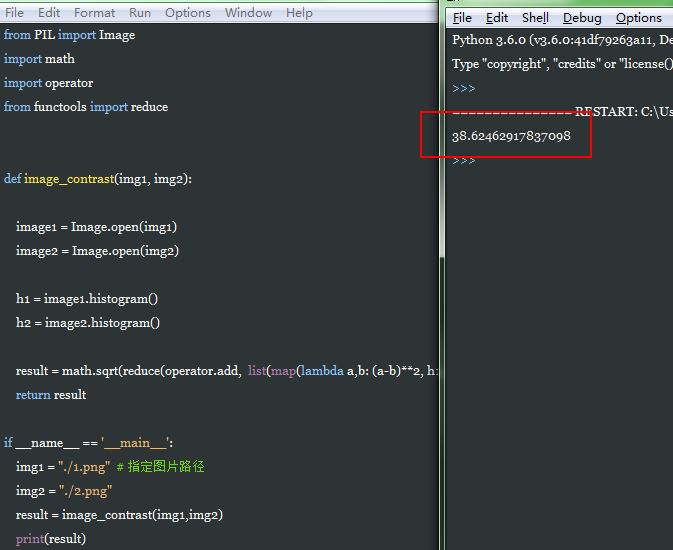# 用python实现对比两张图片的不同

更新时间：2018年02月05日 11:11:48   作者：botoo我要评论

```from PIL import Image
from PIL import ImageChops
def compare_images(path_one, path_two, diff_save_location):
"""
比较图片，如果有不同则生成展示不同的图片
@参数一: path_one: 第一张图片的路径
@参数二: path_two: 第二张图片的路径
@参数三: diff_save_location: 不同图的保存路径
"""
image_one = Image.open(path_one)
image_two = Image.open(path_two)
try:
diff = ImageChops.difference(image_one, image_two)
if diff.getbbox() is None:
# 图片间没有任何不同则直接退出
print("【+】We are the same!")
else:
diff.save(diff_save_location)
except ValueError as e:
text = ("表示图片大小和box对应的宽度不一致，参考API说明：Pastes another image into this image."
"The box argument is either a 2-tuple giving the upper left corner, a 4-tuple defining the left, upper, "
"right, and lower pixel coordinate, or None (same as (0, 0)). If a 4-tuple is given, the size of the pasted "
"image must match the size of the region.使用2纬的box避免上述问题")
print("【{0}】{1}".format(e,text))
if __name__ == '__main__':
compare_images('1.png',
'2.png',
'我们不一样.png')``````from PIL import Image
import math
import operator
from functools import reduce
def image_contrast(img1, img2):
image1 = Image.open(img1)
image2 = Image.open(img2)
h1 = image1.histogram()
h2 = image2.histogram()
result = math.sqrt(reduce(operator.add, list(map(lambda a,b: (a-b)**2, h1, h2)))/len(h1) )
return result
if __name__ == '__main__':
img1 = "./1.png" # 指定图片路径
img2 = "./2.png"
result = image_contrast(img1,img2)
print(result)```http://pillow.readthedocs.org/en/latest/index.html